Courses

# NCERT Solutions(Part - 2) - Understanding Elementary Shapes Class 6 Notes | EduRev

## Class 6 : NCERT Solutions(Part - 2) - Understanding Elementary Shapes Class 6 Notes | EduRev

The document NCERT Solutions(Part - 2) - Understanding Elementary Shapes Class 6 Notes | EduRev is a part of the Class 6 Course Mathematics (Maths) Class 6.
All you need of Class 6 at this link: Class 6

Exercise 5.4
Ques 1: What is the measure of
(i) a right angle?
(ii) a straight angle?
Ans: (i) 90o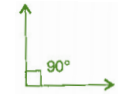(ii) 180o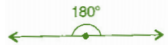Ques 2: Say True or False:
(a) The measure of an acute angle < 90.
(b) The measure of an obtuse angle < 90.
(c) The measure of a reflex angle > 180.
(d) The measure of on complete revolution = 360.
(e) If m∠A = 53o and m∠B = 35o then m∠A > m∠B.
Ans: (a) True
(b) False
(c) True
(d) True
(e) True

Ques 3: Write down the measure of:
(a) some acute angles
(b) some obtuse angles
(give at least two examples of each)
Ans: (a) 35o, 20o
(b) 110o, 135o

Ques 4: Measure the angles give below, using the protractor and write down the measure: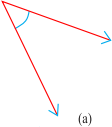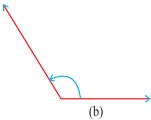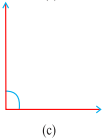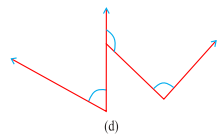Ans: (a) 40o
(b) 130o
(c) 90o
(d) 60o

Ques 5: Which angle has a large measure? First estimate and then measure: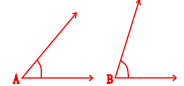Measure of angle A = ?
Measure of angle B = ?
Ans: ∠B has larger measure. ∠A = 40o and ∠B = 65o

Ques 6: From these two angles which has larger measure? Estimate and then confirm by measuring them:
Ans: Second angle has larger measure.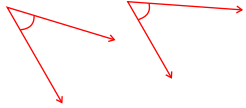Ques 7: Fill in the blanks with acute, obtuse, right or straight:
(a) An angle whose measure is less than that of a right angle is ________________.
(b) An angle whose measure is greater than that of a right angle is ________________.
(c) An angle whose measure is the sum of the measures of two right angles is ________________.
(d) When the sum of the measures of two angles is that of a right angle, then each one of them is ________________.
(e) When the sum of the measures of two angles is that of a straight angle and if one of them is acute then the other should be ________________.
Ans: (a) acute angle
(b) obtuse angle
(c) straight angle
(d) acute angle
(e) obtuse angle

Ques 8: Find the measure of the angle shown in each figure. (First estimate with your eyes and then find the actual measure with a protractor).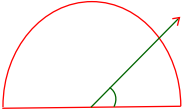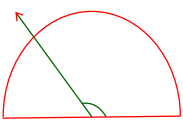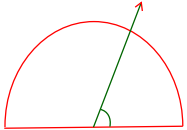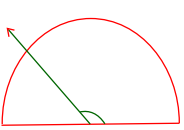Ans: (i) 30o
(ii) 120o
(iii) 60o
(iv) 150o

Ques 9: Find the angle measure between the hands of the clock in each figure: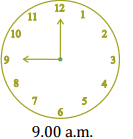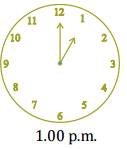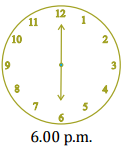Ans: (i) 90 (Right angle)
(ii) 30 (Acute angle)
(iii) 180 (Straight angle)

Ques 10: Investigate:
In the given figure, the angle measure 30. Look at the same figure through a magnifying glass. Does the angle becomes larger? Does the size of the angle change?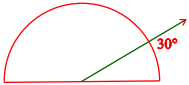Ans: No, the measure of angle will be same.

Ques 11: Measure and classify each angle: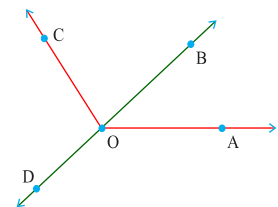Angle Measure Type ∠AOB ∠AOC ∠BOC ∠DOC ∠DOA ∠DOB

Ans:

 Angle ∠AOB ∠AOC ∠BOC ∠DOC ∠DOA ∠DOB Measure 40° 130° 90° 90° 140° 180° Type Acute Obtuse Right Right Obtuse Straight

Exercise 5.5
Ques 1: Which of the following are models for perpendicular lines:
(a) The adjacent edges of a table top.
(b) The lines of a railway track.
(c) The line segments forming the letter ‘L’.
(d) The letter V.
Ans: (a) Perpendicular
(b) Not perpendicular
(c) Perpendicular
(d) Not perpendicular

Ques 2: Let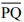be the perpendicular to the line segment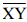. Letandintersect in the point A. What is the measure of ∠PAY?
Ans: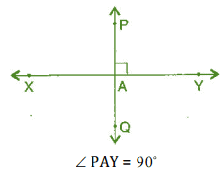Ques 3: There are two “set-squares” in your box. What are the measures of the angles that are formed at their corners? Do they have any angle measure that is common?
Ans: One set-square has 45 ,90 ,45 and other set-square has 60 ,90 ,30 . They have 90 as common angle.

Ques 4: Study the diagram. The line l is perpendicular to line m.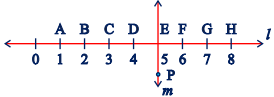(a) Is CE = EG?
(b) Does PE bisect CG?
(c) Identify any two line segments for which PE is the perpendicular bisector.
(d) Are these true?
(i) AC > FG
(ii) CD = GH
(iii) BC < EH
Ans: (a) Yes, both measure 2 units.
(b) Yes, because CE = EG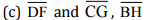(d) (i) True, (ii) True, (iii) True

Exercise 5.6
Ques 1: Name the types of following triangles:
(a) Triangle with lengths of sides 7 cm, 8 cm and 9 cm.
(b) ΔABC with AB = 8.7 cm, AC = 7 cm and BC = 6 cm.
(c) ΔPQR such that PQ = QR = PR = 5 cm.
(d) ΔDEF with m∠D = 90o
(e) ΔXYZ with m∠Y = 90o and XY = YZ
(f) ΔLMN with m∠L = 30o , m∠M = 70o and m∠N = 80o
Ans: (a) Scalene triangle
(b) Scalene triangle
(c) Equilateral triangle
(d) Right-angled triangle
(e) Isosceles right-angled triangle
(f) Acute-angled triangle

Ques 2: Match the following:

 Measure of Triangle Types of Triangle (i) 3 sides of equal length (a) Scalene (ii) 2 sides of equal length (b) Isosceles right angle (iii) All sides are of different length (c) Obtuse angle (iv) 3 acute angles (d) Right angle (v) 1 right angle (e) Equilateral (vi) 1 obtuse angle (f) Acute angle (vii) 1 right angle with two sides of equal length (g) Isosceles

Ans: (i) → (e)
(ii) → (g)
(iii) → (a)
(iv) → (f)
(v) → (d)
(vi) → (c)
(vii) → (b)

Ques 3: Name each of the following triangles in two different ways: (You may judge the nature of angle by observation)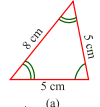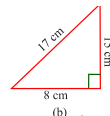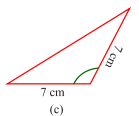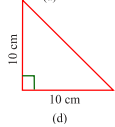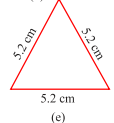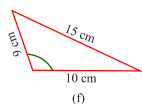Ans: (a) Acute angled triangle and Isosceles triangle
(b) Right-angled triangle and scalene triangle
(c) Obtuse-angled triangle and Isosceles triangle
(d) Right-angled triangle and Isosceles triangle
(e) Equilateral triangle and acute angled triangle
(f) Obtuse-angled triangle and scalene triangle

Ques 4: Try to construct triangles using match sticks. Some are shown here.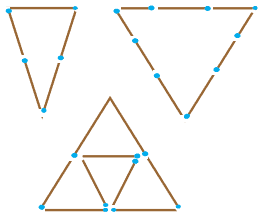Can you make a triangle with:
(a) 3 matchsticks?
(b) 4 matchsticks?
(c) 5 matchsticks?
(d) 6 matchsticks? (Remember you have to use all the available matchsticks in each case)
If you cannot make a triangle, think of reasons for it.
Ans: (a) 3 matchsticks This is an acute angle triangle and it is possible with 3 matchsticks to make a triangle because sum of two sides is greater than third side.
(b) 4 matchsticks This is a square, hence with four matchsticks we cannot make triangle.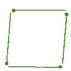(c) 5 matchsticks This is an acute angle triangle and it is possible to make triangle with five matchsticks, in this case sum of two sides is greater than third side.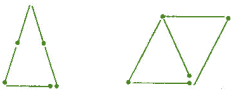(d) 6 matchsticks This is an acute angle triangle and it is possible to make a triangle with the help of 6 matchsticks because sum of two sides is greater than third side.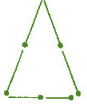Offer running on EduRev: Apply code STAYHOME200 to get INR 200 off on our premium plan EduRev Infinity!

## Mathematics (Maths) Class 6

185 videos|229 docs|43 tests

,

,

,

,

,

,

,

,

,

,

,

,

,

,

,

,

,

,

,

,

,

;